Question

# A long wire carrying 4.50 A of current makes two 90 bends, as shown in the...

A long wire carrying 4.50 A of current makes two 90° bends, as shown in the figure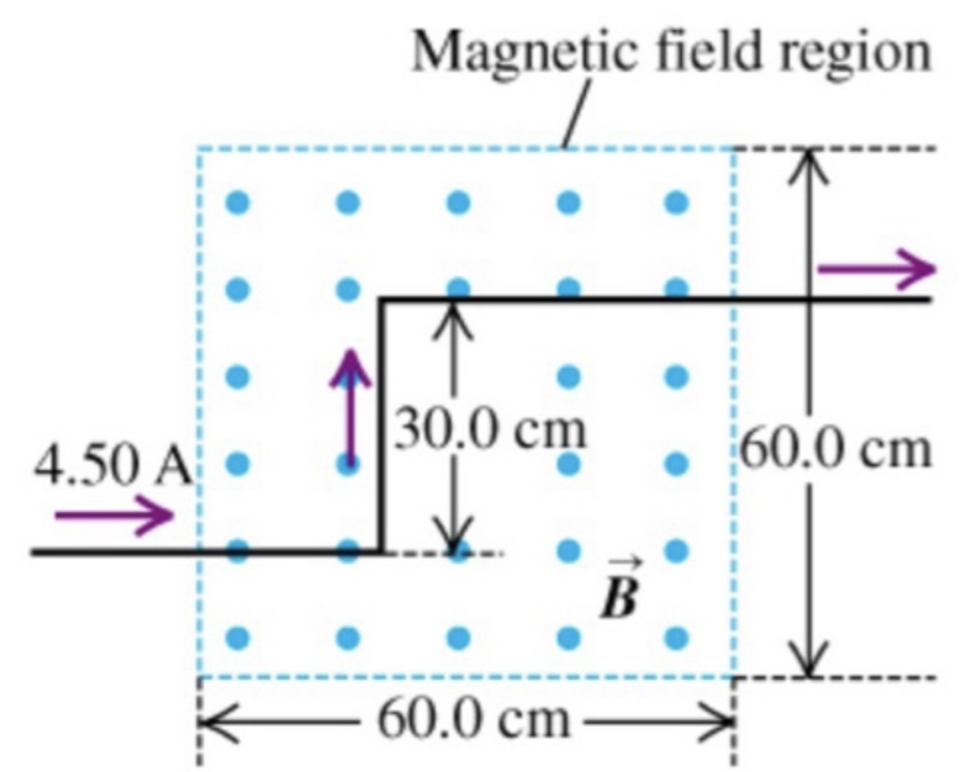The bent part of the wire passes through a uniform 0.238 T magnetic field directed as shown in the figure and confined to a limited region of space.

Part A

Find the magnitude of the force that the magnetic field experts on the the wire.

Part B

Find the direction of the force that the magnetic field experts on the the wire.

The magnetic force on a current carrying conductor $$F=B I L \sin \theta$$

For the horizontal region,

$$F_{H}=0.238 \times 4.5 \times 0.6=0.64 \mathrm{~N}$$

Direction: The magnetic field faces outside the plane of paper, current to the right, hence the force downwards.

For the vertical region,

$$F_{V}=0.238 \times 4.5 \times 0.3=0.32 \mathrm{~N}$$

Direction: The magnetic field faces outside the plane of paper, current to the top, hence the force right.

Total force, $$F=\sqrt{(0.64)^{2}+(0.32)^{2}}=0.72 \mathrm{~N}$$

Direction: Clockwise from the right $$45^{\circ}$$

The final direction angle is wrong...

You take the inverse tan of Fx/Fy

answered by: Abby Bittner

#### Earn Coins

Coins can be redeemed for fabulous gifts.

Similar Homework Help Questions
• ### A long wire carrying 4.50 A of current makes two 90 degree bends, as shown in....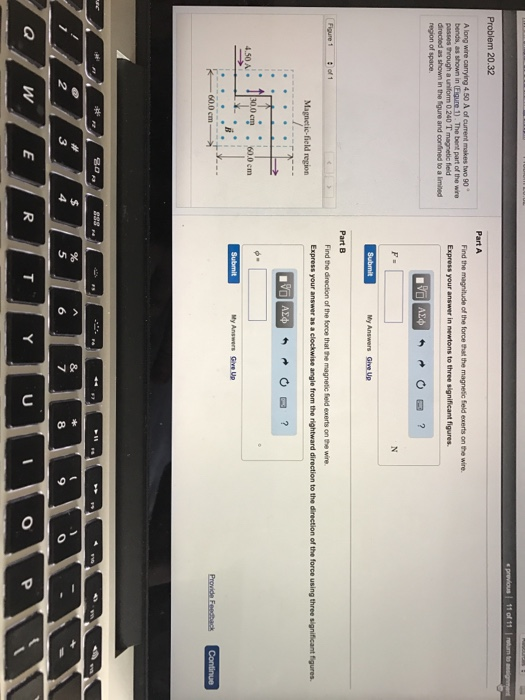A long wire carrying 4.50 A of current makes two 90 degree bends, as shown in. The best part of the wire passes through a uniform 0.240 t magnetic field directed as shown in the figure and confined to a limited of space. Part A Find the magnitude of the force that the magnetic field exerts on the wire Part B Find the direction of the force that the magnetic field exerts on the wire.

• ### A long wire carrying 6.50 A of current makes two bends, as shown in the figure.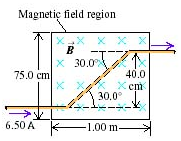A long wire carrying 6.50 A of current makes two bends, as shown in the figure. The bent part of the wire passes through a uniform 0.280 T magnetic field directed as shown in the figure and confined to a limited region of space.A) Find the magnitude of the force that the magnetic field exerts on the wire.B) Find the direction of the force that the magnetic field exerts on the wire.

• ### Problem 4 Magnetic field region Fig. 2 A long wire carrying 4.50 A of current makes...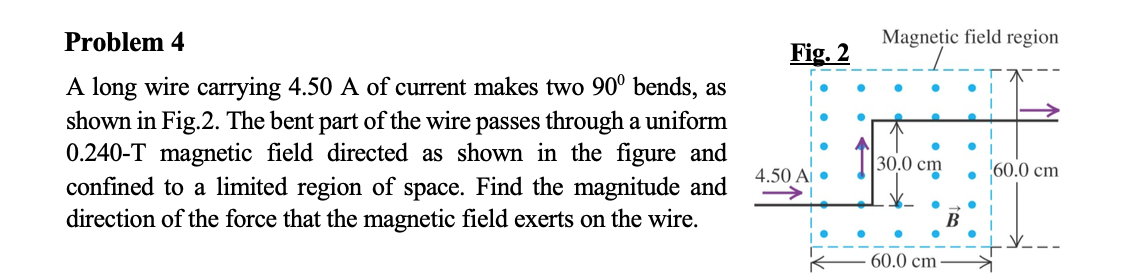Problem 4 Magnetic field region Fig. 2 A long wire carrying 4.50 A of current makes two 90° bends, as shown in Fig.2. The bent part of the wire passes through a uniform 0.240-T magnetic field directed as shown in the figure and confined to a limited region of space. Find the magnitude and direction of the force that the magnetic field exerts on the wire. . ||30.0 cm 60.0 cm 4.5 Y_ - 60.0 cm

• ### ​A long wire carrying 6.50 A of currents makes two bends, as shown in the figure on the right.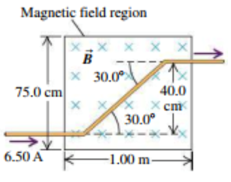A long wire carrying 6.50 A of currents makes two bends, as shown in the figure on the right. The bent part of the wire passes through a uniform 0.280-T magnetic field directed as shown and confined to a limited region of space. a. What are the magnitude and direction of the force exerted on the wire by the magnetic field?b. Now assume that the magnetic field extends 1.00 m to the left and to the right of the region shown...

• ### A long wire carrying a 6.00A current reverses direction by means of two right-angle bends, as...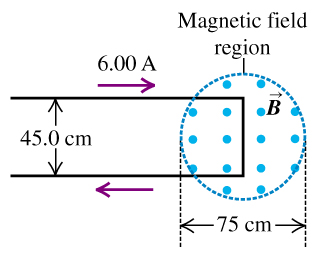A long wire carrying a 6.00A current reverses direction by means of two right-angle bends, as shown in the figure (Figure 1) . The part of the wire where the bend occurs is in a magnetic field of 0.666T confined to the circular region of diameter 75cm, as shown. A) Find the direction of the net force that the magnetic field exerts on this wire. B) Find the magnitude of the net force that the magnetic field exerts on this...

• ### A wire carrying a 31.0 A current bends through a right angle.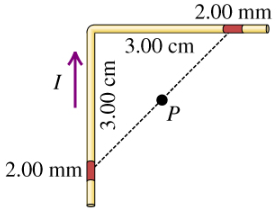Exercise 28.13 - Enhanced - with Solution A wire carrying a 31.0 A current bends through a right angle. Consider two 2.00 mm segments of wire, each 3.00 cm from the bend (Figure 1). Part A Find the magnitude of the magnetic field these two segments produce at point P, which is midway between them. Express your answer with the appropriate units. Part B Find the direction of the magnetic field at point P. out of the page into the page

• ### (​Magnetic Force on a Wire​) A horizontal, current-carrying wire of mass m = 40.0 g is...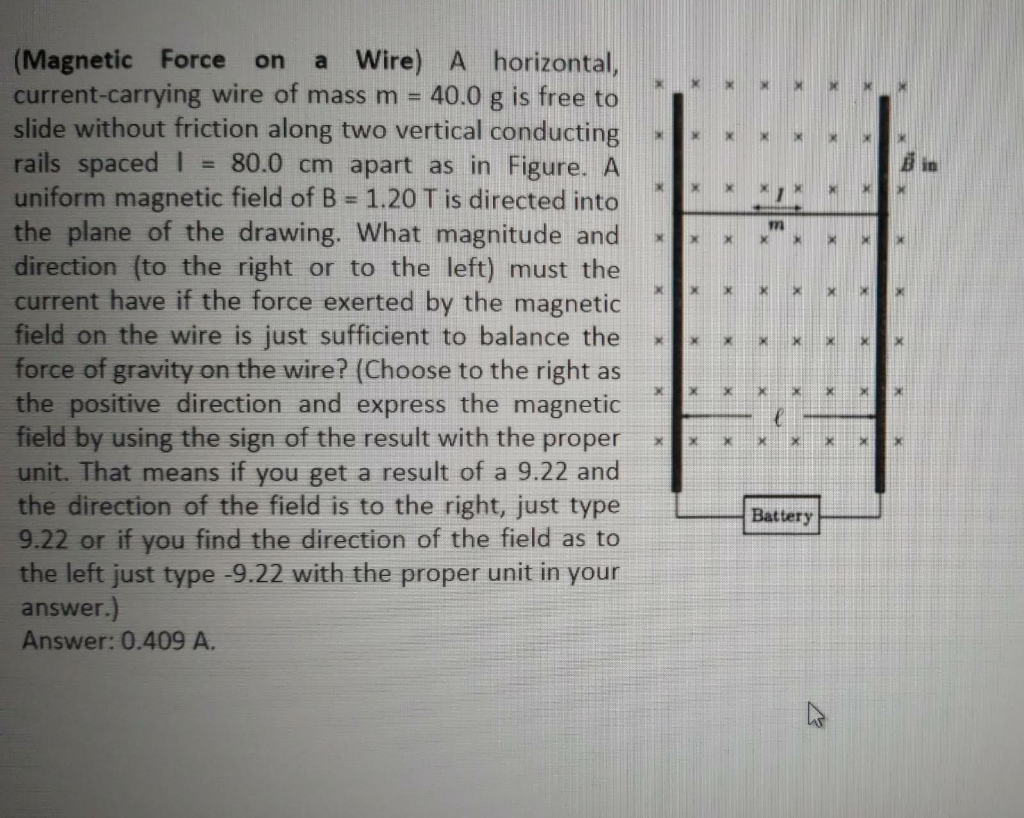(​Magnetic Force on a Wire​) A horizontal, current-carrying wire of mass m = 40.0 g is free to slide without friction along two vertical conducting rails spaced l = 80.0 cm apart as in Figure. A uniform magnetic field of B = 1.20 T is directed into the plane of the drawing. What magnitude and direction (to the right or to the left) must the current have if the force exerted by the magnetic field on the wire is just...

• ### please check to me this An electron (mass 9.11x10 %'kg) has velocity v = (24 i...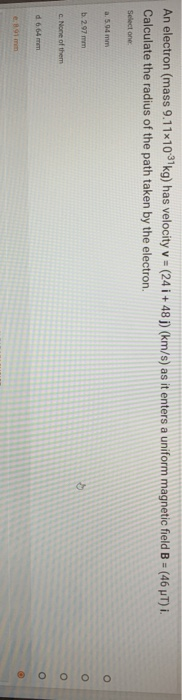please check to me this An electron (mass 9.11x10 %'kg) has velocity v = (24 i +48(km/s) as it enters a uniform magnetic field B = (46 NT) I. Calculate the radius of the path taken by the electron. Select one a. 5.04 b. 297 mm 0 0 0 0 c. None of them d 6.64 MET 8.91 mm Along wire carrying 7.5A of current makes two 90° bends, as shown in Figure. A uniform 0.17 T magnetic field is...

• ### An infinitely long wire carrying a current of 4.5 A is bent at the origin and...

An infinitely long wire carrying a current of 4.5 A is bent at the origin and extends in both the y-direction and x-direction. (In other words, the wire makes a 90 degree angle with the x axis and y axis and has a bend at the origin) Find the magnetic field at the point x = 3 cm and y = 2 cm.